index: click on a letter A B C D E F G H I J K L M N O P Q R S T U V W X Y Z A to Z index index: subject areas numbers & symbols sets, logic, proofs geometry algebra trigonometry advanced algebra & pre-calculus calculus advanced topics probability & statistics real world applications multimedia entrieswww.mathwords.com about mathwords website feedback

Surface Area

The total area of the exterior surface of a solid. Many formulas for the area of a surface are given below.

 For the following tables, h = height of solid s = slant height P = perimeter or circumference of the base l = length of solid B = area of the base r = radius of sphere w = width of solid R = radius of the base a = length of an edge

 Figure Volume Lateral Surface Area Area of the Base(s) Total Surface Area Box (also called rectangular parallelepiped, right rectangular prism) lwh 2lh + 2wh 2lw 2lw + 2lh + 2wh Prism Bh Ph 2B Ph + 2B Pyramid- B - Right PyramidBCylinder Bh - 2B - Right Cylinder Bh Ph 2B Ph + 2B Right Circular Cylinder πR2h 2πRh 2πR2 2πRh + 2πR2 Cone- B - Right Circular ConeπRs or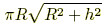πR2 πRs + πR2 or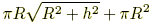Figure Volume Total Surface Area Sphere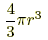Regular Tetrahedron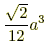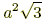Cube (regular hexahedron) a3 6a2 Regular OctahedronRegular Dodecahedron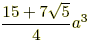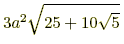Regular Icosahedron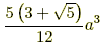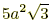For the tables above, h = height of solid s = slant height P = perimeter or circumference of the base l = length of solid B = area of the base r = radius of sphere w = width of solid R = radius of the base a = length of an edge